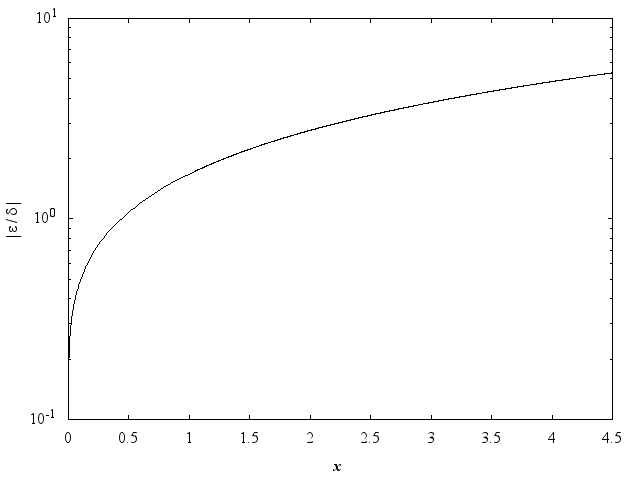NAG Library Function Document

1Purpose

nag_exp_integral (s13aac) returns the value of the exponential integral ${E}_{1}\left(x\right)$.

2Specification

 #include #include
 double nag_exp_integral (double x, NagError *fail)

3Description

nag_exp_integral (s13aac) calculates an approximate value for
 $E1 x = -Ei -x = ∫x∞ e-u u du .$
using Chebyshev expansions, where $x$ is real. For $x<0$, the real part of the principal value of the integral is taken. The value ${E}_{1}\left(0\right)$ is infinite, and so, when $x=0$, nag_exp_integral (s13aac) exits with an error and returns the largest representable machine number.
For $0,
 $E1x=yt-ln⁡x=∑′rarTrt-ln⁡x,$
where $t=\frac{1}{2}x-1$.
For $x>4$,
 $E1x=e-xxyt=e-xx∑′rarTrt,$
where $t=-1.0+\frac{14.5}{\left(x+3.25\right)}=\frac{11.25-x}{3.25+x}$.
In both cases, $-1\le t\le +1$.
For $x<0$, the approximation is based on expansions proposed by Cody and Thatcher Jr. (1969). Precautions are taken to maintain good relative accuracy in the vicinity of ${x}_{0}\approx -0.372507\dots \text{}$, which corresponds to a simple zero of Ei($-x$).
nag_exp_integral (s13aac) guards against producing underflows and overflows by using the argument ${x}_{\mathrm{hi}}$; see the Users' Note for your implementation for the value of ${x}_{\mathrm{hi}}$. To guard against overflow, if $x<-{x}_{\mathrm{hi}}$ the function terminates and returns the negative of the largest representable machine number. To guard against underflow, if $x>{x}_{\mathrm{hi}}$ the result is set directly to zero.
Abramowitz M and Stegun I A (1972) Handbook of Mathematical Functions (3rd Edition) Dover Publications
Cody W J and Thatcher Jr. H C (1969) Rational Chebyshev approximations for the exponential integral Ei$\left(x\right)$ Math. Comp. 23 289–303

5Arguments

1:    $\mathbf{x}$doubleInput
On entry: the argument $x$ of the function.
Constraint: $-{x}_{\mathrm{hi}}\le {\mathbf{x}}<0.0$ or ${\mathbf{x}}>0.0$.
2:    $\mathbf{fail}$NagError *Input/Output
The NAG error argument (see Section 3.7 in How to Use the NAG Library and its Documentation).

6Error Indicators and Warnings

NE_ALLOC_FAIL
Dynamic memory allocation failed.
See Section 2.3.1.2 in How to Use the NAG Library and its Documentation for further information.
NE_INTERNAL_ERROR
An internal error has occurred in this function. Check the function call and any array sizes. If the call is correct then please contact NAG for assistance.
See Section 2.7.6 in How to Use the NAG Library and its Documentation for further information.
NE_NO_LICENCE
Your licence key may have expired or may not have been installed correctly.
See Section 2.7.5 in How to Use the NAG Library and its Documentation for further information.
NE_REAL_ARG_LE
On entry, ${\mathbf{x}}=0.0$ and the function is infinite.
The evaluation has been abandoned due to the likelihood of overflow. The argument ${\mathbf{x}}<-{x}_{\mathrm{hi}}$.

7Accuracy

Unless stated otherwise, it is assumed that $x>0$.
If $\delta$ and $\epsilon$ are the relative errors in argument and result respectively, then in principle,
 $ε≃ e-x E1 x ×δ$
so the relative error in the argument is amplified in the result by at least a factor ${e}^{-x}/{E}_{1}\left(x\right)$. The equality should hold if $\delta$ is greater than the machine precision ($\delta$ due to data errors etc.) but if $\delta$ is simply a result of round-off in the machine representation, it is possible that an extra figure may be lost in internal calculation and round-off.
The behaviour of this amplification factor is shown in the following graph:Figure 1
It should be noted that, for absolutely small $x$, the amplification factor tends to zero and eventually the error in the result will be limited by machine precision.
For absolutely large $x$,
 $ε∼xδ=Δ,$
the absolute error in the argument.
For $x<0$, empirical tests have shown that the maximum relative error is a loss of approximately $1$ decimal place.

8Parallelism and Performance

nag_exp_integral (s13aac) is not threaded in any implementation.

None.

10Example

The following program reads values of the argument $x$ from a file, evaluates the function at each value of $x$ and prints the results.

10.1Program Text

Program Text (s13aace.c)

10.2Program Data

Program Data (s13aace.d)

10.3Program Results

Program Results (s13aace.r)

© The Numerical Algorithms Group Ltd, Oxford, UK. 2017